N1H111SM's Miniverse

# RL(3) - Model-Free Control

2019/08/15 Share# Preface

• MDP model is unknown but can be sampled.
• MDP model is known but it is computationally infeasible to use directly, except through sampling.

On-policy learning 和 Off-policy learning是两种学习的方法，前者在和环境的交互中直接进行学习，也就是当前的policy和当前的sample是对应的；而后者能够从另一个不同的policy中去进行Evaluation和参数的更新。

# Generalized Policy Iteration

MC for On-policy Q Evalution

• Initialize $N(s,a)=0, G(s,a)=0, Q^\pi(s,a)=0, \forall s \in S, \forall a \in A$
• Loop
• Using policy $\pi$ sample episode $i=s_{i,1}, a_{i,1},r_{i,1},s_{i,2}, a_{i,2},r_{i,2}, \dots, s_{i,T_i}$
• $G_{i,t}=r_{i,t}+\gamma r_{i,t+1}+ \gamma^2 r_{i,t+2}+ \dots + \gamma^{T_i-1}r_{i,T_i}$
• Foreach (state,action) $(s,a)$ visited in episode $i$
• For first or every time $t$ that $(s,a)$ is visited in episode $i$
• $N(s,a)=N(s,a)+1, G(s,a)=G(s,a)+G_{i,t}$
• Update estimate $Q^\pi(s,a)=G(s,a)/N(s,a)$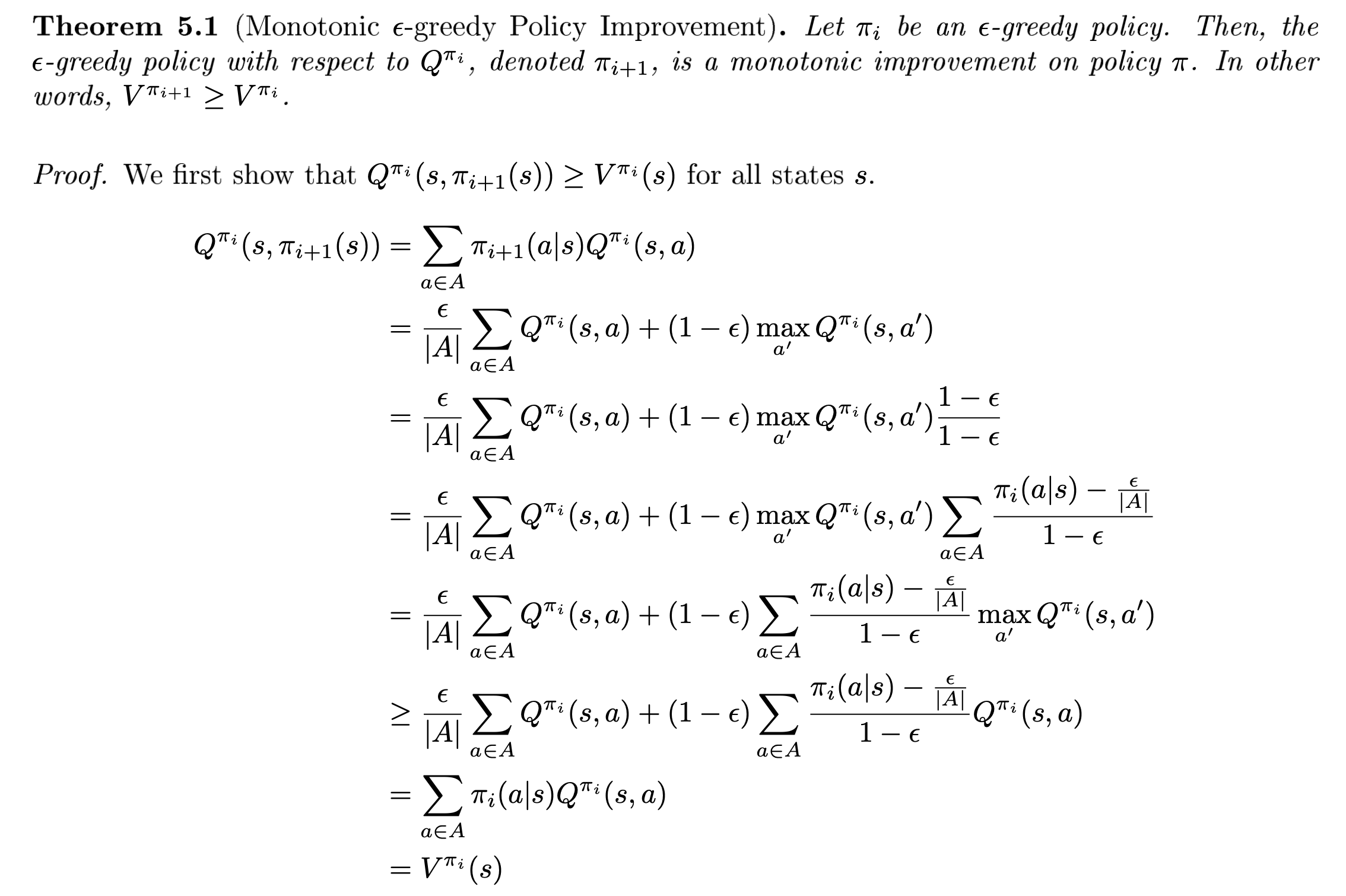Greedy in the Limit of Infinite Exploration (GLIE)

$\epsilon$-greedy策略是能够让每次策略更新依旧保持单调增长的一种方式。而我们可以对这个策略进行适当的refine从而让这种更新策略保证收敛。GLIE的定义由以下两个条件组成：

# Monte Carlo Online Control# Temporal Difference Methods for Control

## SARSA

SARSA算法的伪代码如下图所示，和MC方法的核心变化在于TD方法不需要等一个episode完结才能够进行更新，而是根据下一个step的观察可以直接进行更新，其依据就是利用Bellman方程的estimation：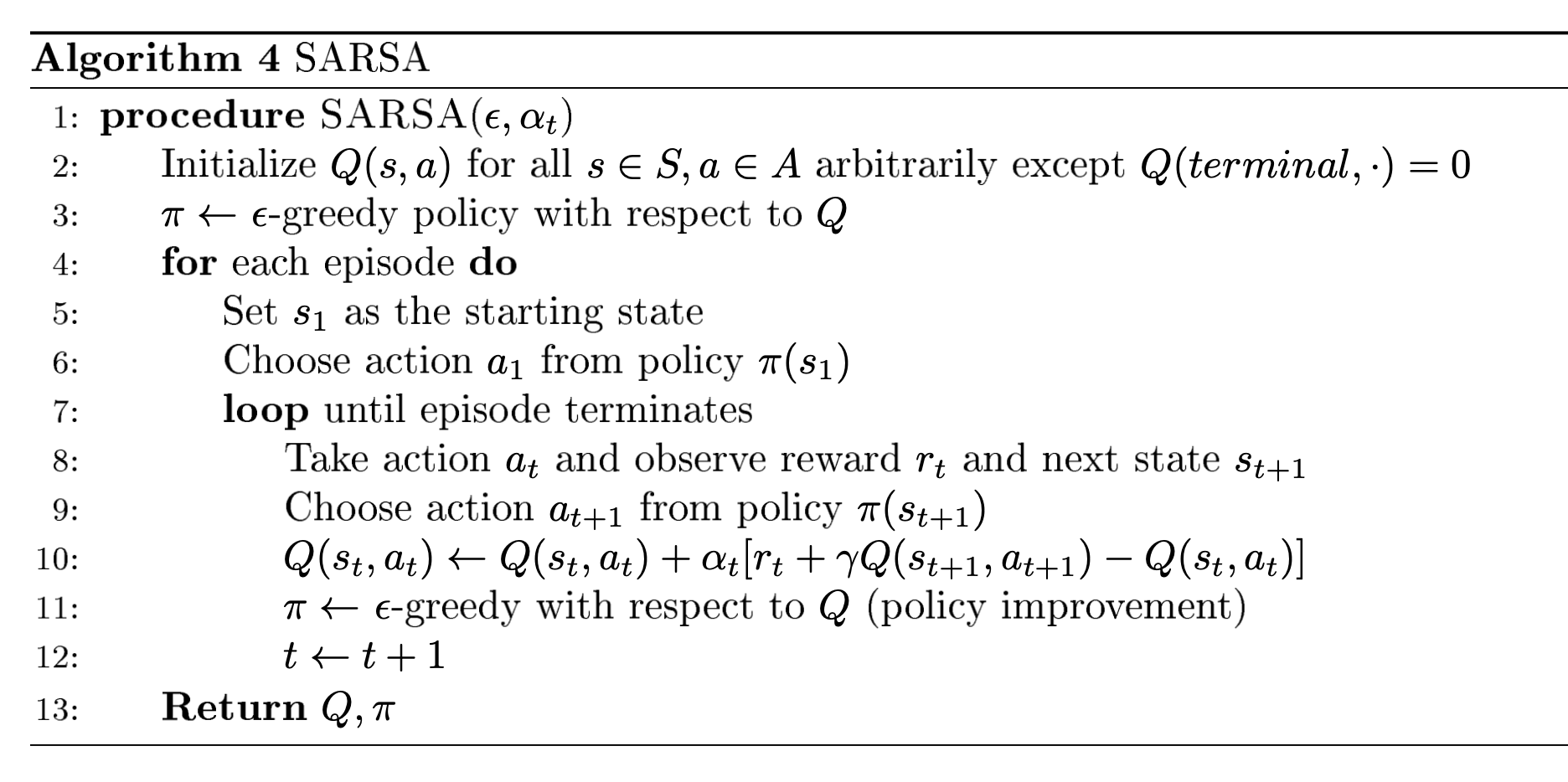SARSA for finite-state & finite-action MDPs 在以下两个条件成立时能够保证收敛到最优的action-value：

• 策略序列$\pi_{t}(a | s)$满足上面所提及的GLIE条件
• 步长$\alpha_t$满足Robbins-Munro序列要求：
• $\sum_{t=1}^{\infty} \alpha_{t}=\infty$
• $\sum_{t=1}^{\infty} \alpha_{t}^{2}<\infty$

## Q-Learning

Q-Learning也是一个Temporal Difference的方法，和SARSA相比，Q-Leaning不需要从policy当中sample出确切的action，而是更新时采用当前时间步最大期望的action-state值进行更新，因此Q-Learning也被认为是一种off-policy的算法。虽然理论上Q-Learning能够确保收敛到和SARSA一样的最优值，但初始化的状态也依旧会产生一些影响。总体来说，optimistic initialization会比较有帮助。以下是两种算法更新函数的比较。

• SARSA：$Q\left(s_{t}, a_{t}\right) \leftarrow Q\left(s_{t}, a_{t}\right)+\alpha\left(\left(r_{t}+\gamma Q\left(s_{t+1}, a_{t+1}\right)\right)-Q\left(s_{t}, a_{t}\right)\right)$
• Q-Learning：$Q\left(s_{t}, a_{t}\right) \leftarrow Q\left(s_{t}, a_{t}\right)+\alpha\left(\left(r_{t}+\gamma \max _{a^{\prime}} Q\left(s_{t+1}, a^{\prime}\right)\right)-Q\left(s_{t}, a_{t}\right)\right)$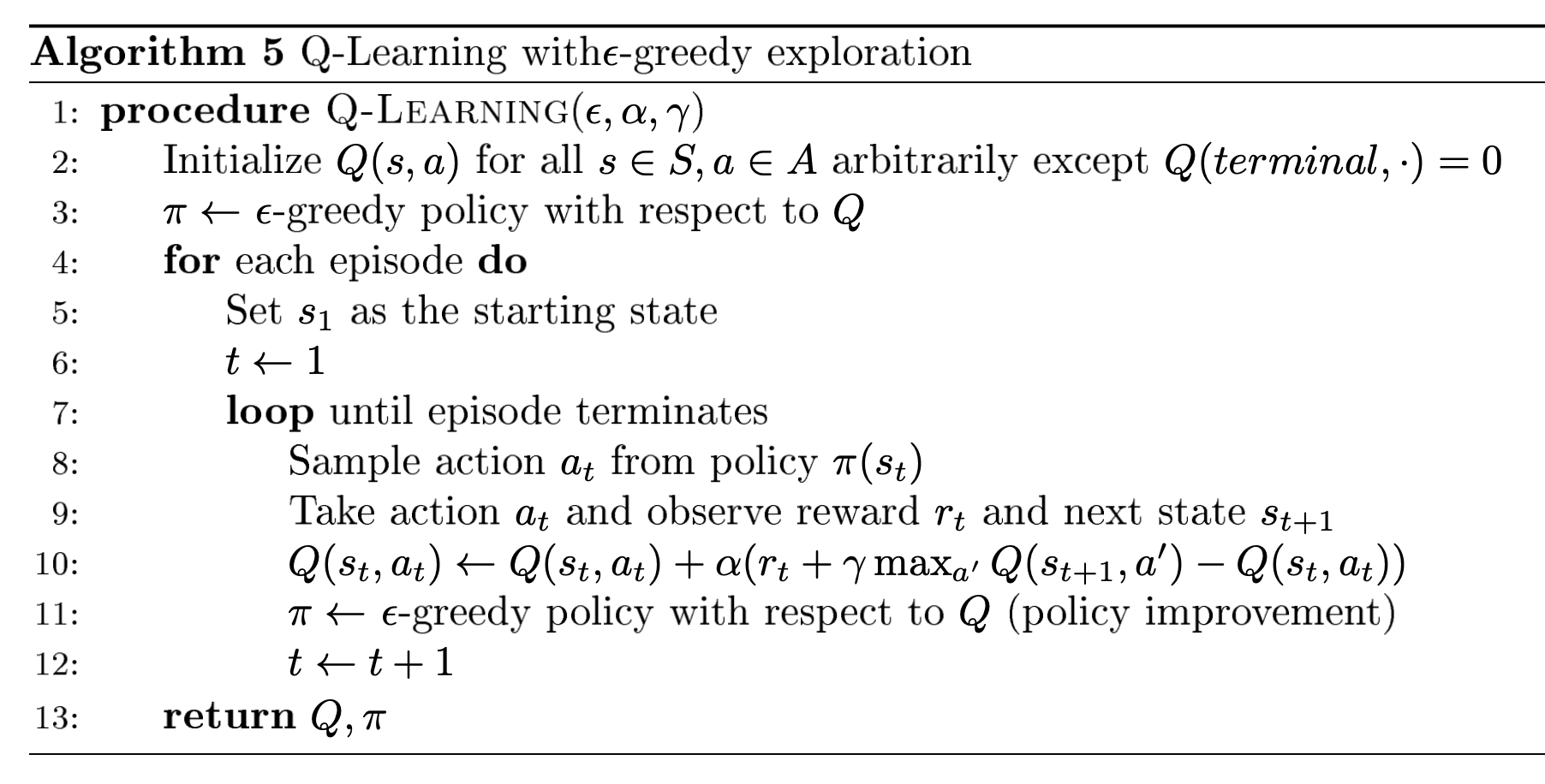# Maximization Bias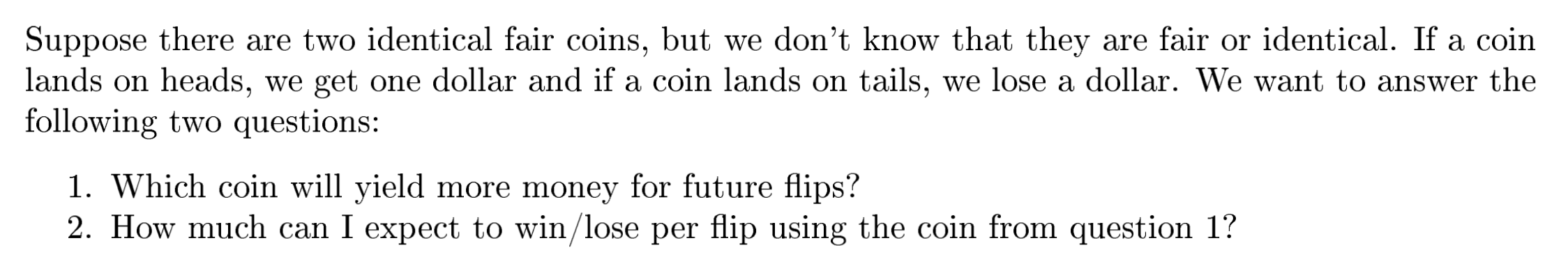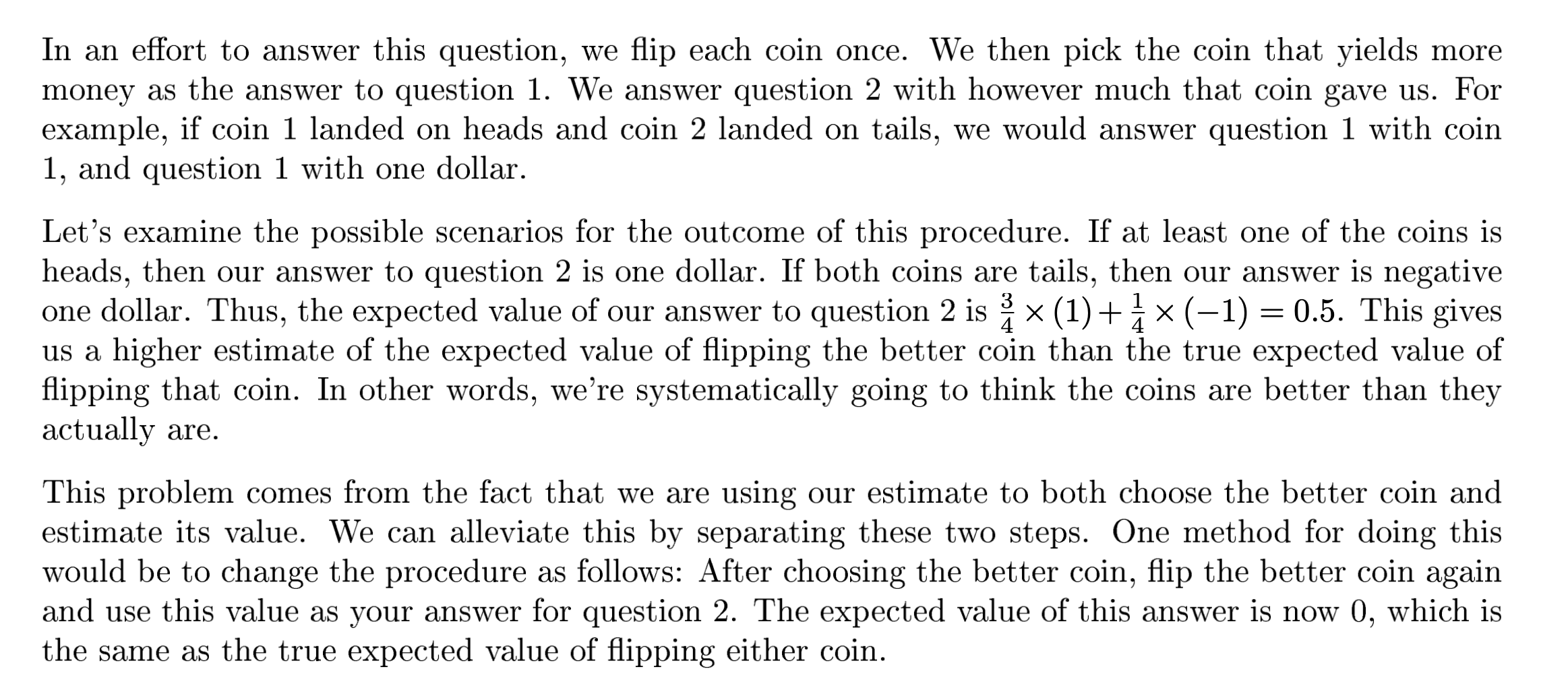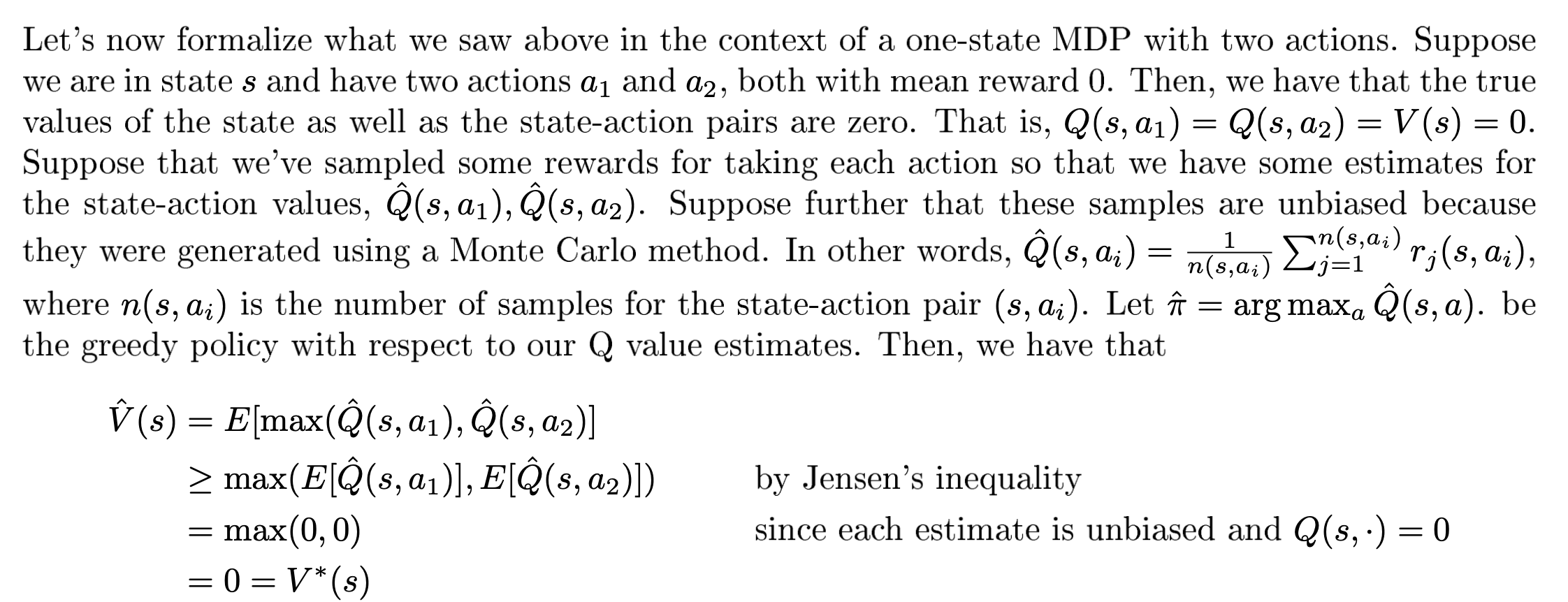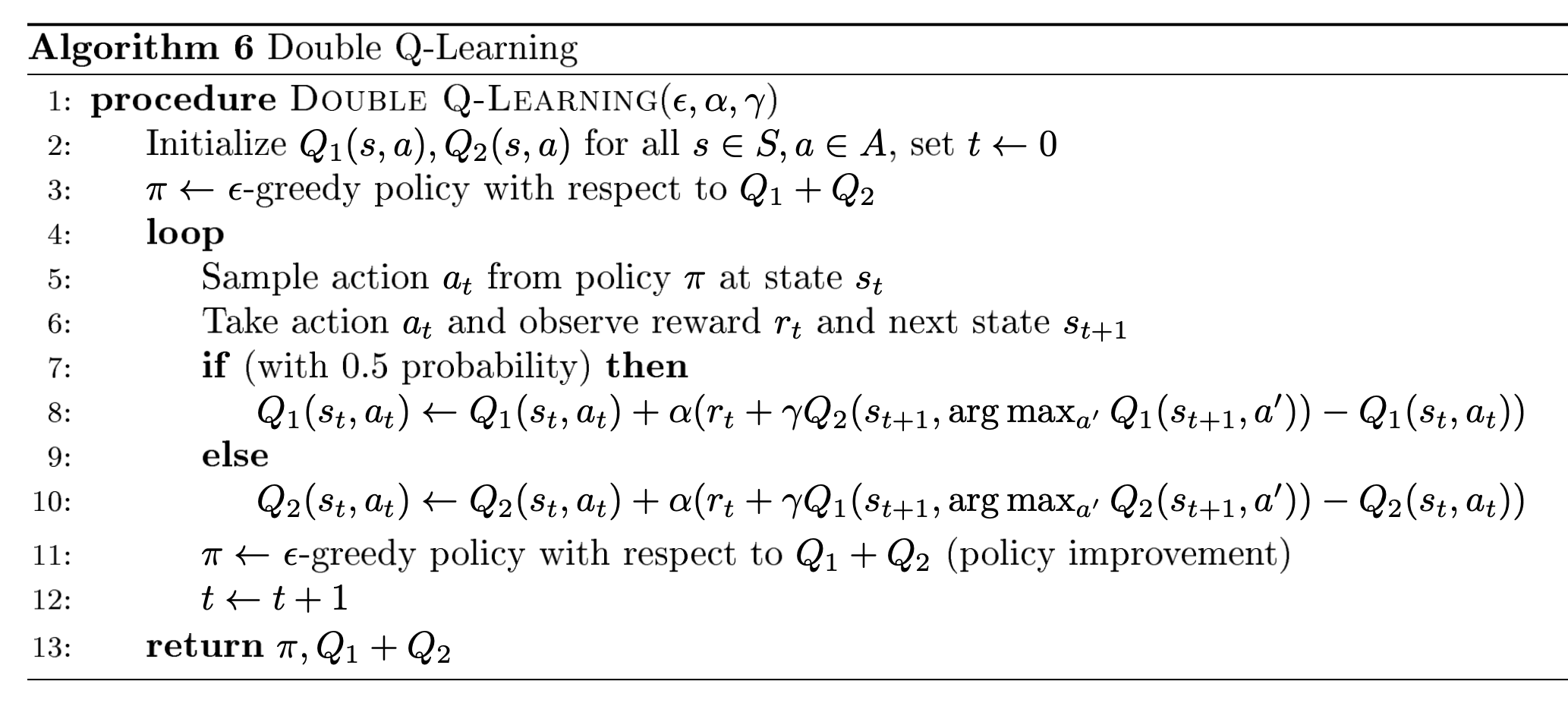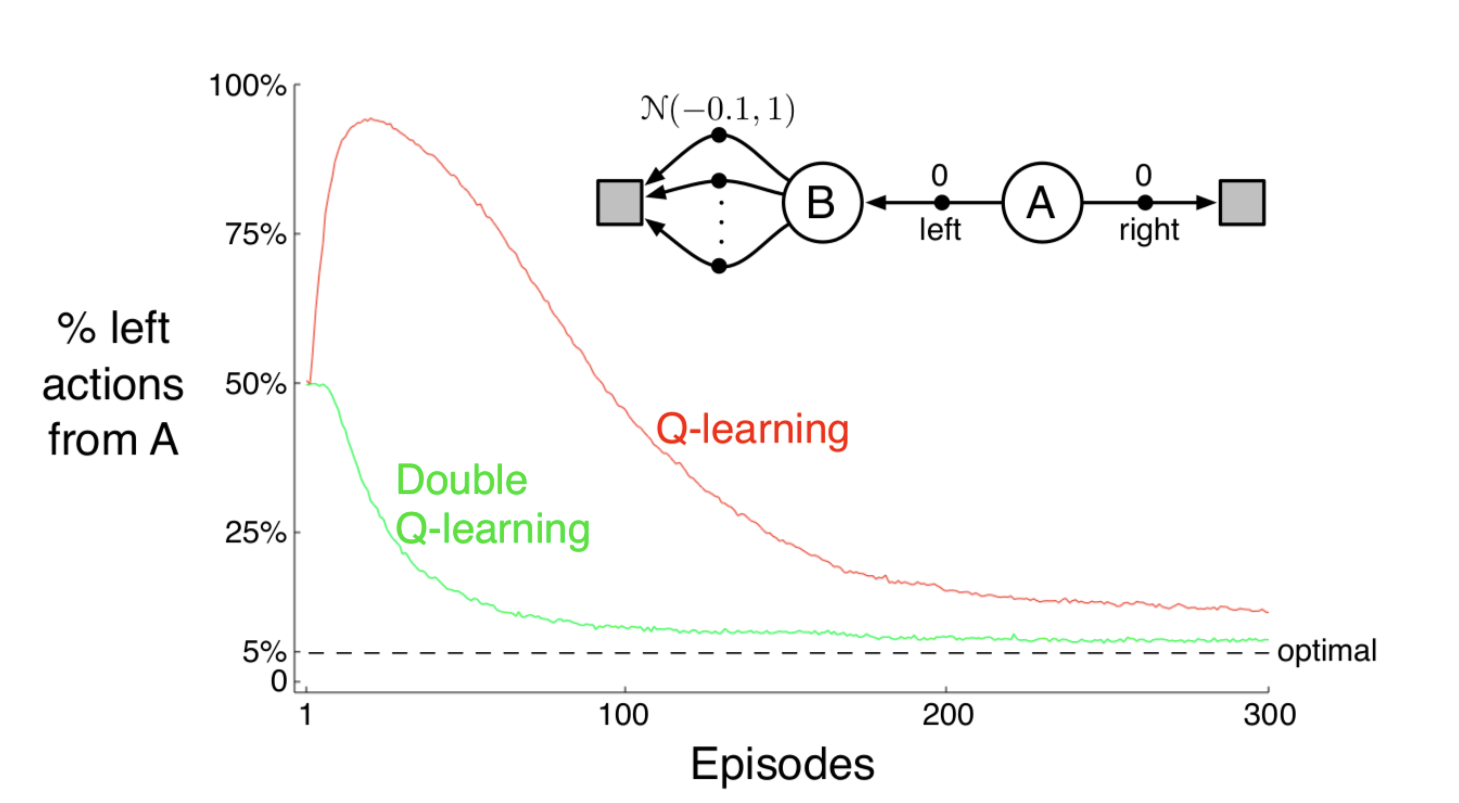What is happening here is that, if an action’s value was overestimated, it will be chosen as the best action, and it’s overestimated value is used as the target. Instead, we can have two different action-value estimates as mentioned in the above equation, and the “best” action can be chosen based on the values of the first action-value estimate and the target can be decided by the second action-value estimate. How do we train though?

Divide the samples into two halves. For the first half, we can use the above equation to train, and for the second half swap the “1” with the “2” in the equation. In practice, data keeps coming into the agent, and thus a coin is flipped to decide if the above equation should be used or the swapped one. How does this help?

Since the samples are stochastic, it is less likely that both the halves of the samples are overestimating the same action. The above method separates two crucial parts of the algorithm, choosing the best action and using the estimate of that action, and hence works well.